Next: SOURCE-RECEIVER RECIPROCITY Up: Mathematical physics in stratified Previous: NUMERICAL MATRIZANTS

# UP- AND DOWNGOING WAVES

We have seen a host of examples of how physical problems in stratified source-free media reduce to the form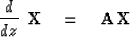(1)
Where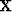is a vector of physical variables and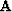is a matrix which depends on z if material properties depend upon z. An important set of new variables in the vector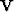is defined by multiplying the vector of physical variablesby a square matrix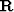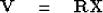(2)
whereis the matrix of row eigenvectors of the matrix.Inverse tois the matrix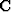of column eigenvectors of.Premultiplying (9-3-2) byand using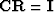we get the inverse relation to (9-3-2) which is useful to find the physical variablesfrom the new variables.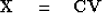(3)
Inserting (9-3-3) into (9-3-1) we obtain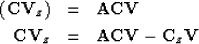(4) (5)
Premultiplying byand using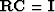we obtain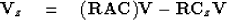(6)
Since we have supposedandto be row and column eigenvector matrices ofwe can replace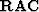by the diagonal matrix of eigenvalues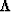,that is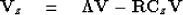(7)
In any region of physical space where the material is homogeneous then, hence,will be independent of z and (9-3-5) will reduce to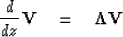(8)
But the only matrix in (9-3-6) is a diagonal matrix, and so the problem for the different variables in the vectordecouples into a separate problem for each component. In wave problems it will be seen to be appropriate to call the components ofupgoing and downgoing wave variables. These variables flow up and down in homogeneous regions without interacting with each other. Let us consider an example.

In Sec. 9-1 we deduced that the matrix first-order differential equation for the acoustic problem in a region of no sources takes the form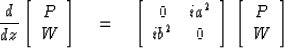(9)
where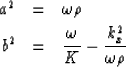(10) (11)

The matrix of column eigenvectorsand the matrix of row eigenvectors of the matrix of (9-3-7) are readily verified to be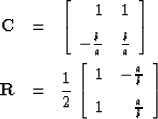(12) (13)
It is also readily verified that the vectors are normalized, namely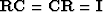and that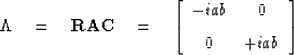The downgoing wave variable D is associated with the iab eigenvalue and the upcoming wave variable U is associated with the -iab. We have definitions for up- and downgoing waves as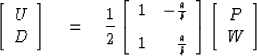(1)

Of course a row eigenvector may contain an arbitrary multiplicative scaling factor if the scaling factor is divided from the corresponding column eigenvector. This means that the definition (9-3-12a) is not unique. As it happens, the present scale factors give the up- and downgoing waves the physical dimensions of P. The physical variables P and W are found from U and D by the inverse relation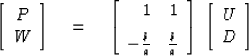(2)
from which we see that the pressure P is the downgoing wave plus the upcoming wave and the vertical velocity is b/a times the difference. Equation (9-3-5) governing the propagation of U and D is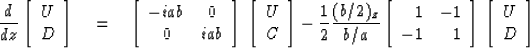(13)

In any region of space where b/a is not a function of z we are left with the simple uncoupled equations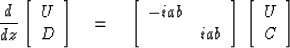(14)

Strictly, to justify the definitions of U and D as up- and downgoing waves we will have to be sure that the downgoing solution takes the form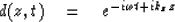(15)
where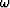and kz must agree in sign so that constant phase is maintained as both z and t increase. The opposite sign must apply to U. In other words kz = ab must take the sign of.To see that this happens we take the square root of the product of (9-3-8) and (9-3-9).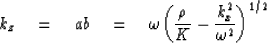(16)
For vertically propagating waves we have kx = 0 so that kz = ab specializes to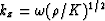.Substituting this value into (9-3-15), we see that the phase angle of the exponential is constant if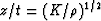,making it clear that the material's intrinsic velocity is given by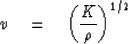(17)
Reference to Figure 9-1 shows that the anglebetween the vertical and a ray is defined by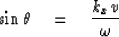(18)
Inserting (9-3-17) and (9-3-18) into (9-3-16) we obtain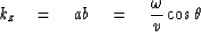(19)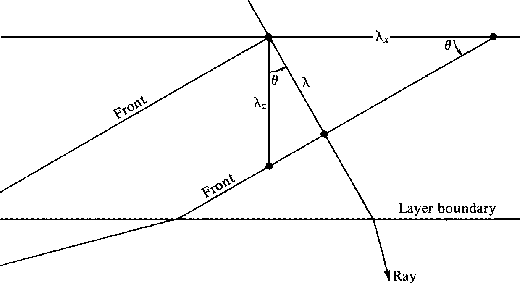9-1
Figure 1
Rays and wavefronts in a layer. The wavelength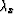seen on the x axis and the wavelength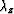seen on the z axis are both greater than the wavelengthseen along the ray. Clearly,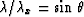and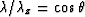so the spatial frequencies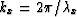and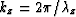satisfy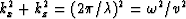, which, besides being the Pythagorean theorem (since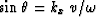), is the Fourier transform of the wave equation. Snell's law that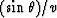is the same from layer to layer is thus equivalent to saying that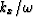is the same in each layer. That the spatial frequency kx is the same constant in each layer is essential to the satisfaction of continuity conditions at the layer interfaces.

The time function (9-3-15) is complex. To get a real time function the expression (9-3-15) must be summed or integrated to include both positive and negative frequencies. Then, as we saw in the chapters on time series analysis, we must have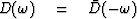.

The quantity b/a will turn out to be the material's characteristic admittance Y. Taking the square root of the ratio of (9-3-9) over (9-3-8) we have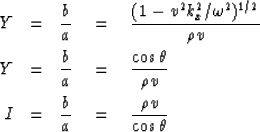(20) (21) (22)

We shall now verify that this definition of impedance is the same as the one in the previous chapter. To do this we take a careful look at the matrizant to cross a layer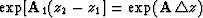.By Sylvester's theorem we have for the matrizant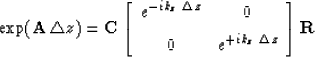(23)
The matrizant relates the wave variables at the top z1 of a layer to those at the bottom z2. Thus (9-3-23) enables us to write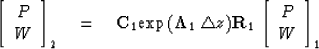(24)
Equation (9-3-24) which seems to have jumped at us from the mysteries of Sylvester's theorem actually has a simple interpretation. Starting on the right, we interpret the multiplication of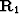into the P and W variables as a conversion to up- and downgoing variables. Then the multiplication by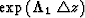carries these across the layer and the multiplication by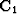converts back to P and W variables which are continuous crossing an interface. Multiplying (9-3-24) through by R2 and noting (9-3-11) and (9-3-12) we have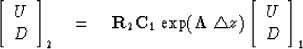(25)
In (9-3-25) we have now defined the up- and downgoing waves just beneath the interface as we did in the previous chapter. We should now be able to recognize the matrix as having the same form. It is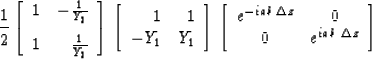(26)
Defining the Z transform variable by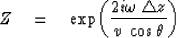Now we recognize that the travel time across the layer is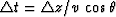. The layer matrix (9-3-26) is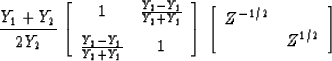(27)
which may be compared to the matrix of (8-2-4) namely,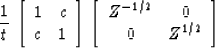establishing that the definition Y = b/a has led to the familiar definition of reflection coefficient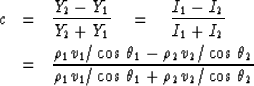(28)

## EXERCISES:

1. Redefine the eigenvectors so that W + D + U and P = (D - U)/Y. This transformation would be useful if we wanted t = 1 + c to refer to vertical velocity normalized variables instead of pressure variables as in Chap. 8. Deduce changes to all the equations of this section.
2. Write the matrizant which crosses a layer in terms of a, b, and layer thickness h.Next: SOURCE-RECEIVER RECIPROCITY Up: Mathematical physics in stratified Previous: NUMERICAL MATRIZANTS
Stanford Exploration Project
10/30/1997